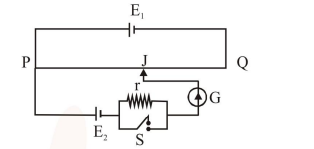# A potentiometer wire PQ of

Question:

A potentiometer wire PQ of $1 \mathrm{~m}$ length is connected to a standard cell $\mathrm{E}_{1}$. Another cell $\mathrm{E}_{2}$ of emf $1.02 \mathrm{~V}$ is connected with a resistance 'r' and switch S (as shown in figure). With switch $S$ open, the null position is obtained at a distance of $49 \mathrm{~cm}$ from Q. The potential gradient in the potentiometer wire is:1. $0.02 \mathrm{~V} / \mathrm{cm}$

2. $0.04 \mathrm{~V} / \mathrm{cm}$

3. $0.01 \mathrm{~V} / \mathrm{cm}$

4. $0.03 \mathrm{~V} / \mathrm{cm}$

Correct Option: 1

Solution:

Balancing length is measured from $P .$

So $\quad 100-49=51 \mathrm{~cm}$

$\mathrm{E}_{2}=\phi \times 51$

Where $\phi=$ Potential gradient

$1.02=\phi \times 51$

$\phi=0.02 \mathrm{~V} / \mathrm{cm}$